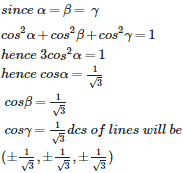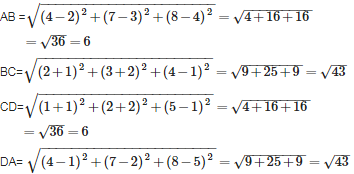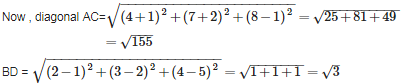# Introduction to Three Dimensional Geometry - Online Test

Q1. The distance of the point ( 3, 4, 5) from X- axis is
Explaination / Solution:

The distance of the point ( 3, 4, 5) from X- axis is

let L be the foot of perpendicular from the point ( 3, 4, 5) to X axis ,then coordinate of L will be (3,0,0)       [ because on X axis y and z coordinate are zero]

then distance of the point ( 3, 4, 5) from X- axis i.e. from L (3,0,0) is given by

Q2. The direction cosines of X -axis are
Explaination / Solution:

As we know that if a lines makes angles b and c with X-axis , Y-axis and Z-axis respectively then direction cosines are given by  cos a , cos b ,cos c >

In our case line is X-axis itself which we know makes angle of 0${}^{\circ }$ , 90${}^{\circ }$ , 90${}^{\circ }$ with  X-axis , Y-axis and Z-axis respectively then direction cosine will be

<cos 0${}^{\circ }$ , cos 90${}^{\circ }$, cos 90${}^{\circ }$>

= < 1 0 0 >

Q3. The medians of a triangle are concurrent at the point called
Explaination / Solution:

The centroid is the point of concurrency of the medians of the triangle.it is a point of centre of gravity of triangle

Q4. The direction cosines of any normal to the XY plane are
Explaination / Solution:

Concept used - Parellel lines have same direction cosines

Explanation We know Z axis is perpendicular to XY plane and direction cosines of Z axis are <0,0,1>

and as  any normal to XY plane will be parallel to Z axis and parellel lines have same direction cosines so answer is <0,0,1>

Q5. How many lines through the origin make equal angles with the coordinate axes ?
Explaination / Solution:hence the lines will be 8

Q6. the numbers 3, 4 , 5 can be
Explaination / Solution:

the numbers 3, 4 , 5 can be direction ratio of any line these not satisfying any other option

Q7. If the direction cosines of a straight line are < k , k , k > , then what will be the value of k
Explaination / Solution:

We know that if  l , m , n are direction cosines of a lines then l2+m2 +n2= 1

so,  k2 + k2 +k2 = 1

3 k2 = 1

k2 = 1/3

taking squareroot on both sides , we have

Q8. The lines, which do not lie in the same plane, are called ?
Explaination / Solution:

Lines which lie in same plane can be parallel, coincident or intersecting. Skew lines are those lines which lie in different planes and never meet to each other

Q9. The radius of the sphere through the points ( 4 ,3 , 0 ) , ( 0 , 4 , 3 ) ,( 0 , 5 , 0 ) and ( 4 , 0 , 3 ) is
Explaination / Solution:

we know that general equation of sphere is

since sphere passing through the points  ( 4 ,3 , 0 ) , ( 0 , 4 , 3 ) ,( 0 , 5 , 0 ) and ( 4 , 0 , 3 ) so putting these values one by one in given equation , we get

16+ 9+ 8g + 6f + c=0    =>8g +6f +c = -25  -------------1)

16 +9 +8f +6h +c=0    =>8f + 6h +c= -25   -----------2)

25 + 10f +c  = 0       -----------------------3)

16+ 9+ 8g + 6h +c=0    =>8g +6h +c= -25  -------------4)

by 2) - 4 ) we have  f = g

using this in 1) we have 16f +c = -25 -------5)

Now solving 3) and 5) we get f=0

using value of f  we have g= 0 , h= 0 , c= -25

Q10. The points ( 4,7 ,8) ,( 2, 3,4 ),( - 1 , -2 , 1 ) and (1 , 2, 5 ) are the vertices of a
Explaination / Solution:

The vertices of a quadrilateral are A( 4,7 ,8) , B( 2, 3,4 ) ,C (- 1 , -2 , 1 ) and D(1 , 2, 5 )Here opposite sides of quadrilateral ABCD are equal , so  it may be parallelogram or rectangleHere length of diagonals AC and BD are different so ABCD is a parrallelogram